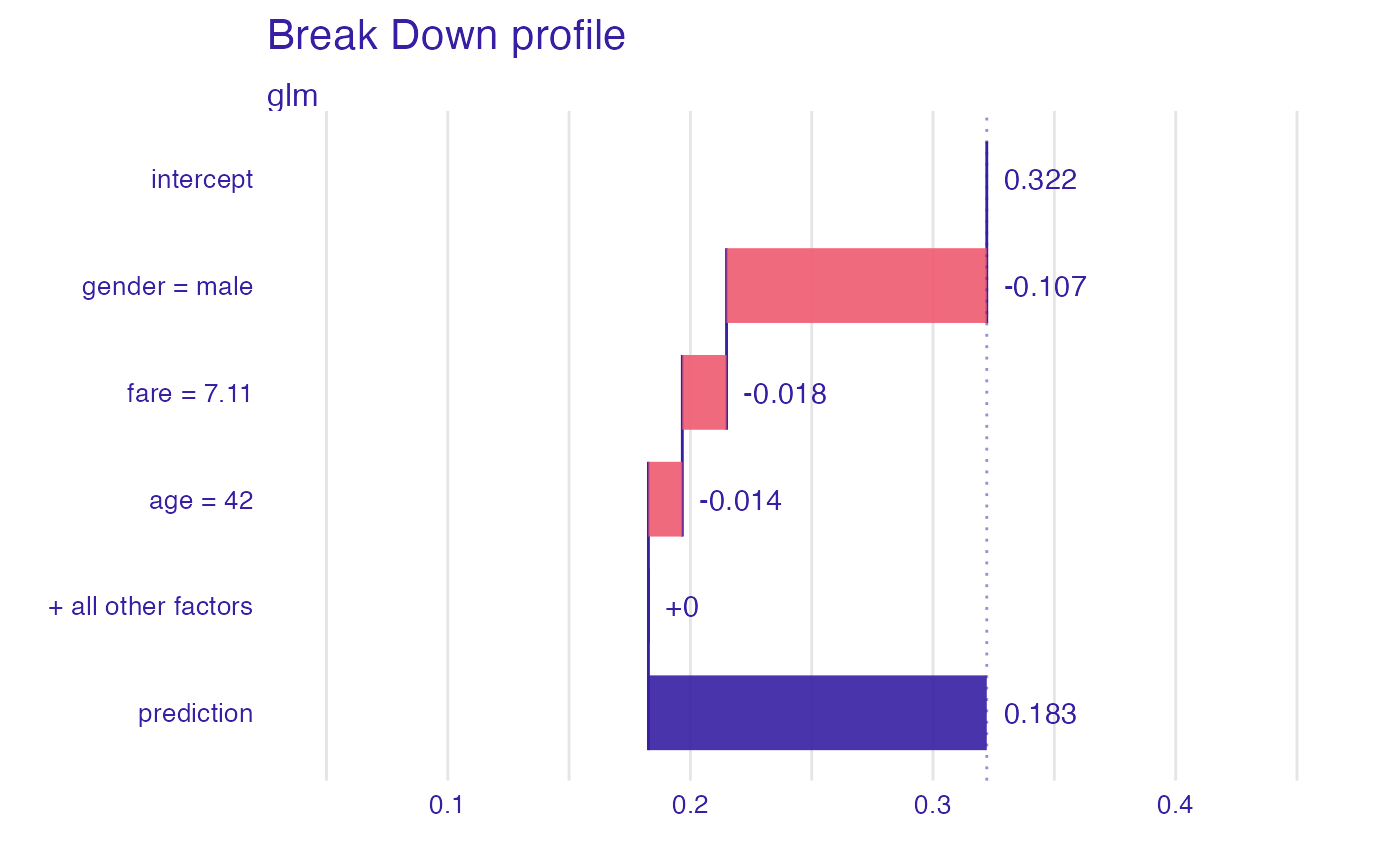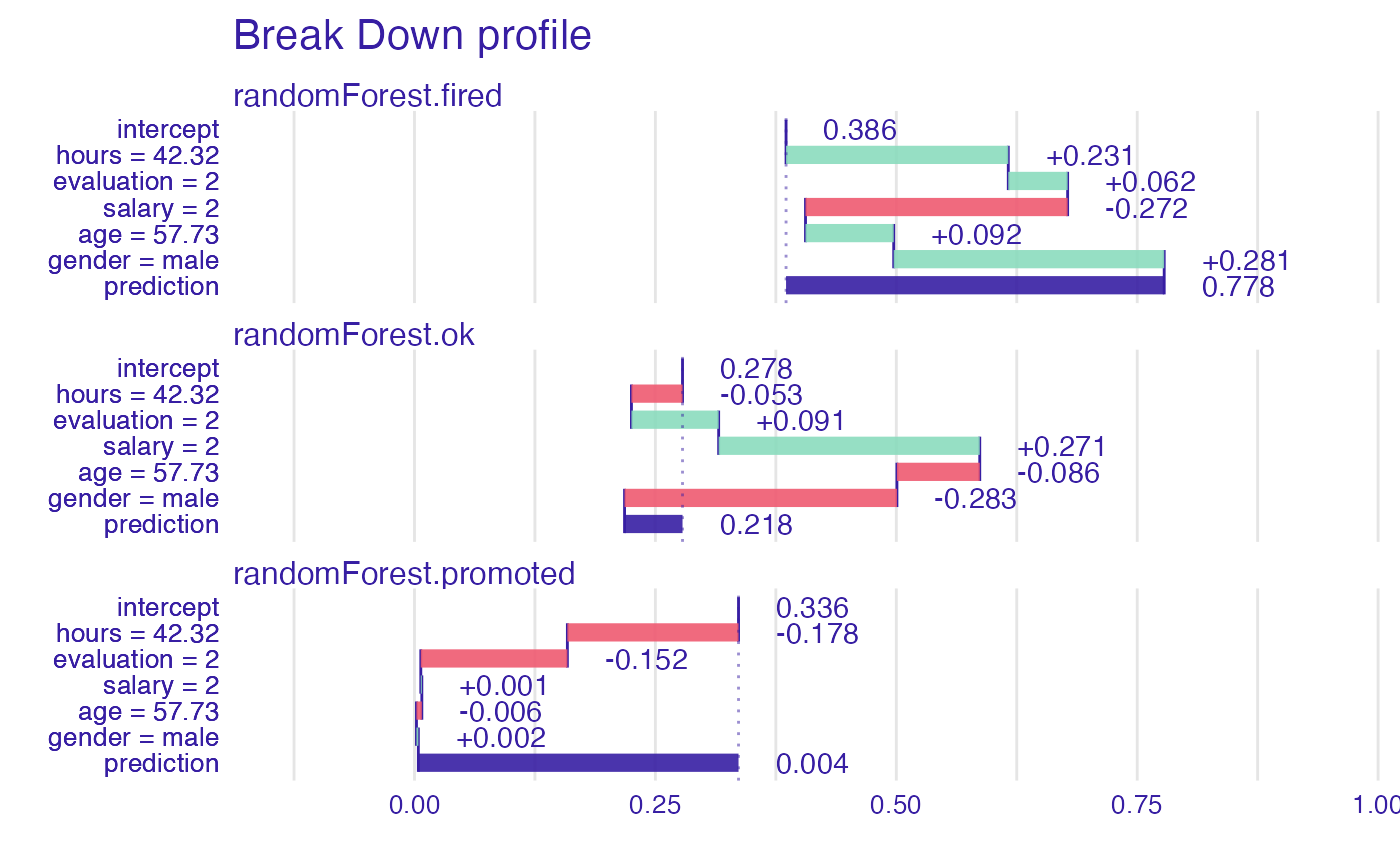This function finds Variable Attributions via Sequential Variable Conditioning. It calls either local_attributions for additive attributions or local_interactions for attributions with interactions.

break_down(x, ..., interactions = FALSE)

# S3 method for explainer
break_down(x, new_observation, ..., interactions = FALSE)

# S3 method for default
break_down(
x,
data,
predict_function = predict,
new_observation,
keep_distributions = FALSE,
order = NULL,
label = class(x),
...,
interactions = FALSE
)

## Arguments

x

an explainer created with function explain or a model.

...

parameters passed to local_* functions.

interactions

shall interactions be included?

new_observation

a new observation with columns that correspond to variables used in the model.

data

validation dataset, will be extracted from x if it is an explainer.

predict_function

predict function, will be extracted from x if it's an explainer.

keep_distributions

if TRUE, then distribution of partial predictions is stored and can be plotted with the generic plot().

order

if not NULL, then it will be a fixed order of variables. It can be a numeric vector or vector with names of variables.

label

name of the model. By default it is extracted from the 'class' attribute of the model.

## Value

an object of the break_down class.

local_attributions, local_interactions

## Examples

library("DALEX")
#> Welcome to DALEX (version: 2.3.0).
#> Find examples and detailed introduction at: http://ema.drwhy.ai/
#> Additional features will be available after installation of: ggpubr.
#> Use 'install_dependencies()' to get all suggested dependencies
library("iBreakDown")
set.seed(1313)
model_titanic_glm <- glm(survived ~ gender + age + fare,
data = titanic_imputed, family = "binomial")
explain_titanic_glm <- explain(model_titanic_glm,
data = titanic_imputed,
y = titanic_imputed\$survived,
label = "glm")
#> Preparation of a new explainer is initiated
#>   -> model label       :  glm
#>   -> data              :  2207  rows  8  cols
#>   -> target variable   :  2207  values
#>   -> predict function  :  yhat.glm  will be used (  default  )
#>   -> predicted values  :  No value for predict function target column. (  default  )
#>   -> model_info        :  package stats , ver. 4.1.2 , task classification (  default  )
#>   -> predicted values  :  numerical, min =  0.1490412 , mean =  0.3221568 , max =  0.9878987
#>   -> residual function :  difference between y and yhat (  default  )
#>   -> residuals         :  numerical, min =  -0.8898433 , mean =  4.198546e-13 , max =  0.8448637
#>   A new explainer has been created!

bd_glm <- break_down(explain_titanic_glm, titanic_imputed[1, ])
bd_glm
#>                             contribution
#> glm: intercept                     0.322
#> glm: gender = male                -0.107
#> glm: fare = 7.11                  -0.018
#> glm: age = 42                     -0.014
#> glm: class = 3rd                   0.000
#> glm: embarked = Southampton        0.000
#> glm: sibsp = 0                     0.000
#> glm: parch = 0                     0.000
#> glm: survived = 0                  0.000
#> glm: prediction                    0.183
plot(bd_glm, max_features = 3)# \dontrun{
## Not run:
library("randomForest")
#> randomForest 4.7-1
#> Type rfNews() to see new features/changes/bug fixes.
set.seed(1313)
# example with interaction
# classification for HR data
model <- randomForest(status ~ . , data = HR)
new_observation <- HR_test[1,]

explainer_rf <- explain(model,
data = HR[1:1000,1:5])
#> Preparation of a new explainer is initiated
#>   -> model label       :  randomForest  (  default  )
#>   -> data              :  1000  rows  5  cols
#>   -> target variable   :  not specified! (  WARNING  )
#>   -> predict function  :  yhat.randomForest  will be used (  default  )
#>   -> predicted values  :  No value for predict function target column. (  default  )
#>   -> model_info        :  package randomForest , ver. 4.7.1 , task multiclass (  default  )
#>   -> model_info        :  Model info detected multiclass task but 'y' is a NULL .  (  WARNING  )
#>   -> model_info        :  By deafult multiclass tasks supports only factor 'y' parameter.
#>   -> model_info        :  Consider changing to a factor vector with true class names.
#>   -> model_info        :  Otherwise I will not be able to calculate residuals or loss function.
#>   -> predicted values  :  predict function returns multiple columns:  3  (  default  )
#>   -> residual function :  difference between 1 and probability of true class (  default  )
#>   A new explainer has been created!

bd_rf <- break_down(explainer_rf,
new_observation)